# Divisible - Definition with Examples

The Complete K-5 Math Learning Program Built for Your Child

• 30 Million Kids

Loved by kids and parent worldwide

• 50,000 Schools

Trusted by teachers across schools

• Comprehensive Curriculum

Aligned to Common Core

##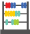Let's learn!

What does divisible mean?
In math, a number is said to be divisible by another number if the remainder is 0.

Divisibility rules are a set of general rules that are often used to determine whether or not a number is evenly divisible by another number.

 Divisibility Rules Example 2: If the number is even or end in 0,2,4, 6 or 8, it is divisible by 2.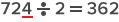3: If the sum of all of the digits is divisible by three, the number is   divisible by 3.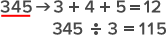4: If the number formed by the last two digits is divisible by 4, the   number is divisible by 4.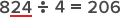5: If the last digit is a 0 or 5, the number is divisible by 5.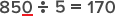6: If a number is divisible by both three and two, it is divisible by 6.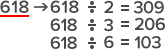7: If the difference of last digit doubled and the rest of the digits is   divisible by seven, the number is divisible by 7.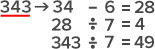8: If the last three digits of a number are divisible by 8, the     number is divisible by 8.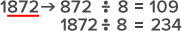9: If the sum of the digits is divisible by nine, the number is   divisible by 9.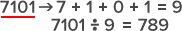10: If the last digit of the number is 0, it is divisible by 10.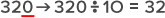Fun Facts Every number is divisible by 1.  When a number is divisible by another number, then it is also divisible by each of the factors of that number. For instance, a number divisible by 6, will also be divisible by 2 and 3.

##Let's sing!

Divisibility rules are good to know,
With them, division is not that slow!
Check the end digits or their sum every time,
To check their divisibility by 2, 3, 5, 10 or 9!

##Let's do it!

Instead of handing out division worksheets to you children, give them a blank sheet of paper and pencil. Write numbers on small pieces of paper, put them in a bowl and then, ask them to pick up chits of the numbers from the bowl, and use the divisibility rules of 2 – 10 to check the divisibility of the numbers.

##Related math vocabulary

Won Numerous Awards & Honors